# Complex Square Root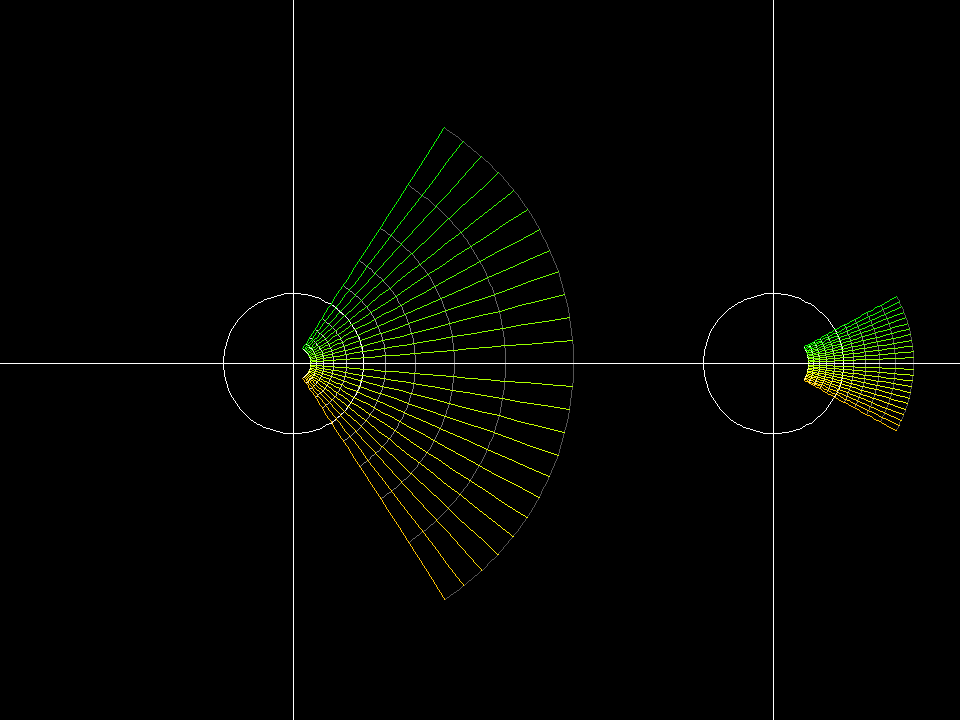square root gauss 001 Every complex number except 0 has two square roots (theese have opposite signs). Therefore one cannot define the square root as a function on the whole complex plane. The standard choice is to define the square root in the Gaussian plane except on the negative real axis. It is defined as a differentiable inverse function of the squaring function. On the negative real axis one defines square_root(-r^2) = + i*r.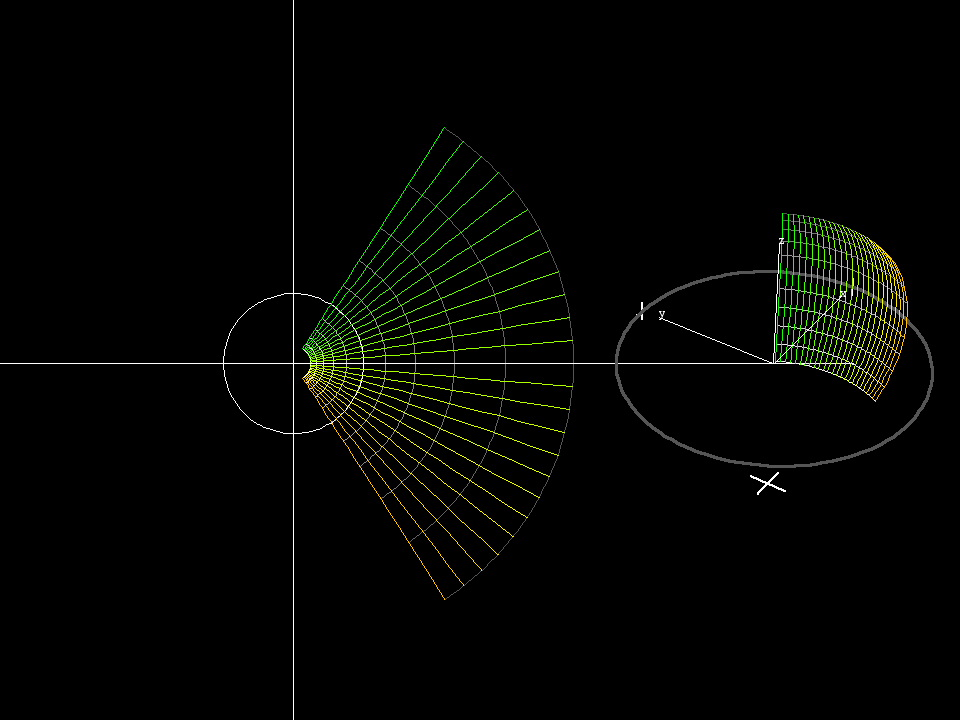square root riemann 001 By applying the stereographic projection we can als view the previous image sequence on the Riemann sphere.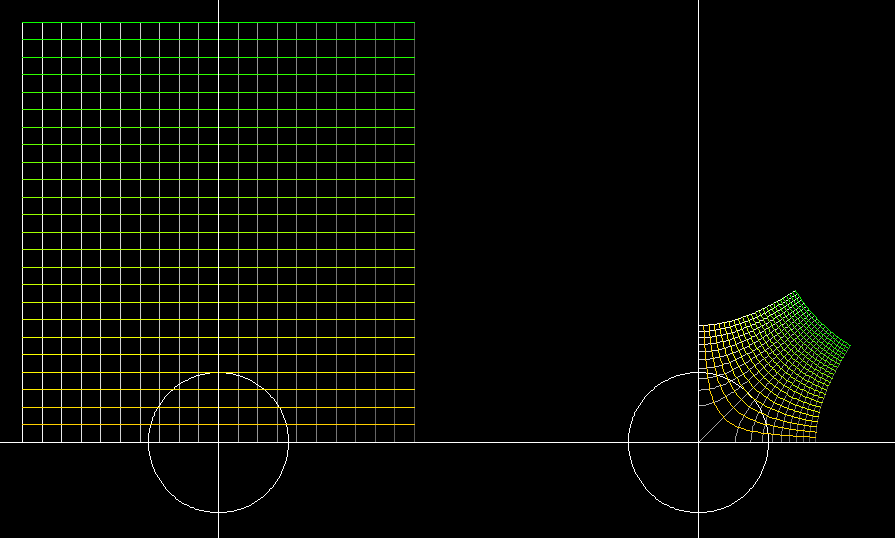square root cartesian gauss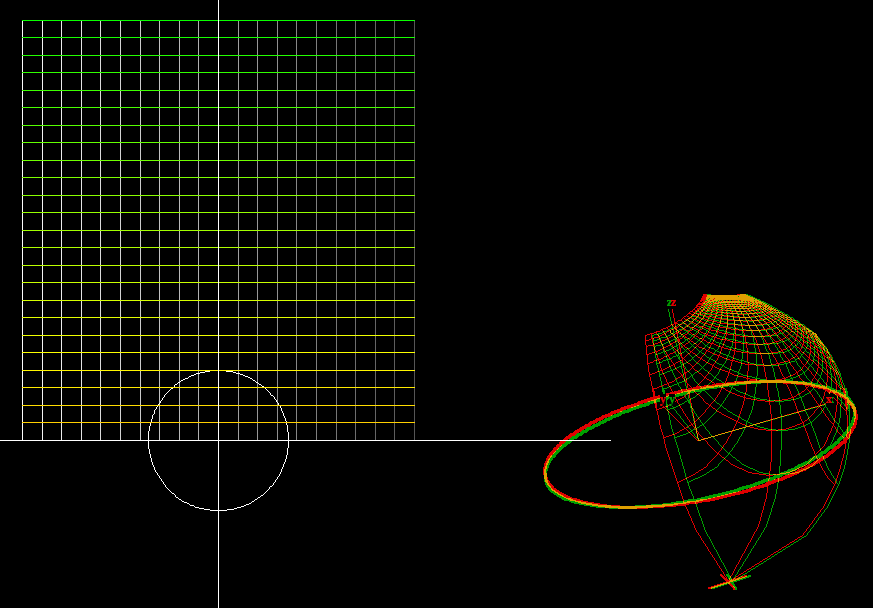The image, under the complex square root, of the previous cartesian grid: viewed in anaglyph on the Riemann sphere.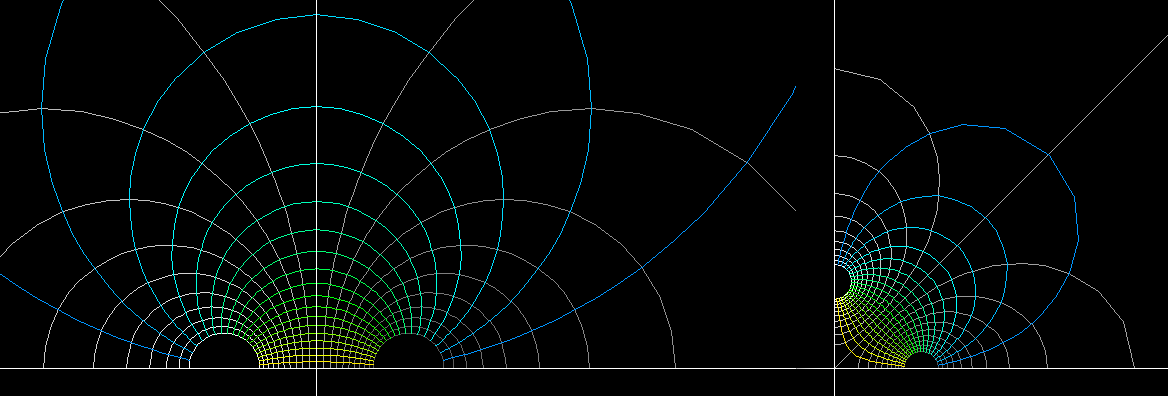The complex square root can be applied to any grid in the left or the upper half plane. The left grid was made with the Moebius transformation z ⟶ (z-1)/(z+1), it moves the polar centers from zero and infinity to +1, -1. The right grid, the image under the complex square root can be reflected in its straight boundary lines to obtain a grid for the whole plane with four polar centers. Such grids are used for the parametrization of minimal surfaces like the 4-noids or the saddle towers.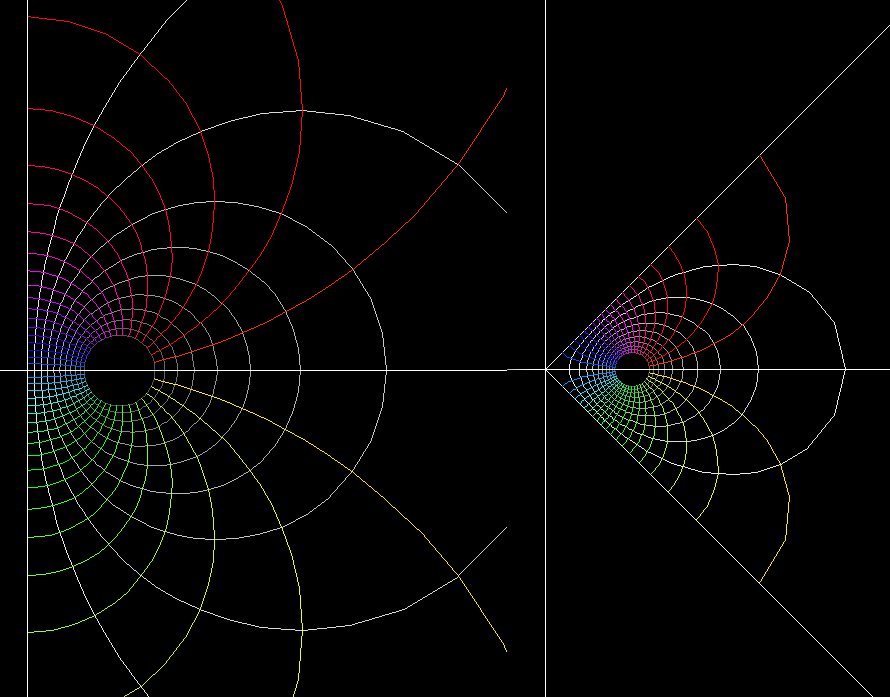SquareRootMoebius2 The complex square root can be applied to any grid in the left or the upper half plane. The left grid was made with the Moebius transformation z ⟶ (z-1)/(z+1), it moves the polar centers from zero and infinity to +1, -1. The right grid, the image under the complex square root can be reflected in its straight boundary lines to obtain a grid for the whole plane with four polar centers. Such grids are used for the parametrization of minimal surfaces like the 4-noids or the saddle towers.

z_sqrt.pdf